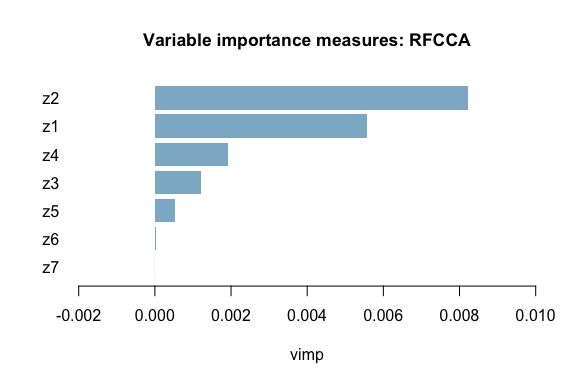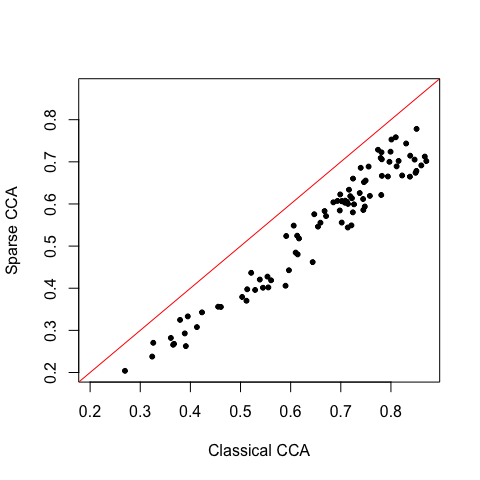# 1 Introduction

This R package implements the Random Forest with Canonical Correlation Analysis (RFCCA) method described in Alakus et al. (2020) <arXiv:2011.11555>. The theoretical details are given in the next section. The last section presents a data analysis using this method.

# 2 Method

## 2.1 Motivation

Multi-view data denotes many kinds of data that provide information about a subject from multiple sources. Integration of multiple feature sets and investigating the relationships between them may help to understand their interactions and obtain more meaningful interpretations. Canonical correlation analysis (CCA) is a multivariate statistical method that analyzes the relationship between two multivariate data sets, $$X$$ and $$Y$$. CCA searches for linear combinations of each of the two data sets, $$Xa$$ and $$Yb$$, having maximum correlation.

The multi-view data collection may also include some subject-related covariates such as age, gender and IQ as one of the views. We want to identify the linear association between two sets of variables, $$X$$ and $$Y$$, but we assume that this association can depend on a third set of subject-related covariates $$Z$$.

## 2.2 Random forest with canonical correlation analysis

We consider the following setting: let $$X$$ and $$Y$$ be two multivariate data sets of dimension $$p$$ and $$q$$, respectively, and let $$Z$$ be a $$r$$-dimensional vector of subject-related covariates. We assume that the canonical correlation between $$X$$ and $$Y$$ depends on $$Z$$. RFCCA uses an unsupervised random forest based on the set of covariates $$Z$$ to find subgroups of observations with similar canonical correlations between $$X$$ and $$Y$$. This random forest consists of many unsupervised decision trees with a specialized splitting criterion $\sqrt{n_Ln_R}*|\rho_L - \rho_R|$ where $$\rho_L$$ and $$\rho_R$$ are the canonical correlation estimations of left and right nodes, and $$n_L$$ and $$n_R$$ are the left and right node sizes, respectively. This splitting rule seeks to increase the canonical correlation heterogeneity. Following the random forest growing, RFCCA builds the Bag of Observations for Prediction (BOP), which is the set of training observations that are in the same terminal nodes as the observation of interest, for a new observation. Then, it applies CCA to the observations in BOP to estimate the canonical correlation of the new observation.

## 2.3 Global significance test

We perform a hypothesis test to evaluate the global effect of the subject-related covariates on distinguishing between canonical correlations. Define the unconditional canonical correlation between $$X$$ and $$Y$$ as $$\rho_{\tiny CCA}(X,Y)$$ which is found by computing CCA with all $$X$$ and $$Y$$, and the conditional canonical correlation between $$X$$ and $$Y$$ given $$Z$$ as $$\rho(X,Y | Z)$$ which is found by RFCCA. If there is a global effect of $$Z$$ on correlations between $$X$$ and $$Y$$, $$\rho(X,Y | Z)$$ should be significantly different from $$\rho_{\tiny CCA}(X,Y)$$. We conduct a permutation test for the null hypothesis $H_0 : \rho(X,Y | Z) = \rho_{\tiny CCA}(X,Y)$ We estimate a p-value with the permutation test. If the $$p$$-value is less than the pre-specified significance level $$\alpha$$, we reject the null hypothesis.

# 3 Data analysis

We will show how to use RFCCA package on a generated data set. The data set has three sets of variables: $$X \in R^{n \times 2}$$, $$Y \in R^{n \times 2}$$ and $$Z \in R^{n \times 7}$$. The sample size ($$n$$) is 300. The correlation between $$X$$ and $$Y$$ depends only on $$Z_1,...,Z_5$$ (i.e. $$Z_6$$ and $$Z_7$$ are noise variables). Firstly, we load the data and split the data into train and test sets:

library(RFCCA)
data(data)

set.seed(2345)
smp <- sample(dim(data$X), round(dim(data$X)*0.7))
train.X <- data$X[smp,] train.Y <- data$Y[smp,]
train.Z <- data$Z[smp,] test.Z <- data$Z[-smp,]

Then, we train the random forest with rfcca().

rf.obj <- rfcca(X = train.X, Y = train.Y, Z = train.Z, ntree = 100)

Before analyzing the prediction results, we have to check first if the the global effect of $$Z$$ is significant by applying the global significance test.

test.obj <- global.significance(X = train.X, Y = train.Y, Z = train.Z,
ntree = 100, nperm = 10)
test.obj$pvalue #>  0 Using 10 permutations, the estimated p-value < 0.05 (where the significance level($$\alpha$$) is 0.05). Hence, we reject the null hypothesis. 10 permutations to compute the p-value is indeed very low. Using 500 permutations, we got a p-value of 0. Higher number of permutations is required to estimate the p-value accurately. However, it should also be noted that higher number of permutations increases the computational time. Since the global effect of $$Z$$ is significant, now we can analyze the predictions. We can get the out-of-bag (OOB) predictions from the grow object. pred.oob <- rf.obj$predicted.oob
head(pred.oob)
#>  0.5263960 0.7352453 0.8860735 0.8399364 0.7000552 0.7289403

Those values represent the OOB canonical correlation predictions for the training observations. The predicted canonical correlations range between 0 and 1 where the closer the values to 1, the stronger the correlation between the canonical variates, $$Xa$$ and $$Yb$$.

We can get the variable importance (VIMP) measures for $$Z$$. VIMP measures reflect the predictive power of subject-related covariates on the estimated correlations.

vimp.obj <- vimp(rf.obj)
vimp <- vimp.obj$importance vimp #> z1 z2 z3 z4 z5 #> 5.557943e-03 8.211117e-03 1.212345e-03 1.917000e-03 5.265072e-04 #> z6 z7 #> 2.502570e-05 -3.598314e-06 Moreover, we can plot the variable importance measures. We know that the correlation between $$X$$ and $$Y$$ depends on $$Z_1,...,Z_5$$. Therefore, it is expected to get higher VIMP measures for $$Z_1,...,Z_5$$ than $$Z_6$$ and $$Z_7$$. plot.vimp(vimp.obj)Finally, we can get canonical correlation predictions for test $$Z$$. pred.obj <- predict(rf.obj, test.Z) pred <- pred.obj$predicted
head(pred)
#>  0.7992133 0.8384541 0.8507449 0.7020309 0.7554645 0.8491357

Those values represent the canonical correlation predictions for the test observations.

Besides the canonical correlation estimations, we can get the estimated canonical coefficients for the $$X$$ and $$Y$$, $$a$$ and $$b$$, respectively.

head(pred.obj$predicted.coef$coefx)
#>               x1           x2
#> [1,] -0.01924032 -0.005634079
#> [2,]  0.02137541  0.008023398
#> [3,]  0.01921067  0.007128489
#> [4,] -0.02172506 -0.010416897
#> [5,] -0.01817261 -0.007220831
#> [6,] -0.01957365 -0.006767873
head(pred.obj$predicted.coef$coefy)
#>                y1          y2
#> [1,] -0.009872529 -0.01711068
#> [2,]  0.017822016  0.01310872
#> [3,]  0.015484555  0.01413854
#> [4,] -0.020101562 -0.01284218
#> [5,] -0.009241092 -0.01731675
#> [6,] -0.015585575 -0.01425509

We can analyse the linear relations between the variables with the entries of the estimated canonical coefficient vectors, $$a$$ and $$b$$. The values under the $$X_1$$ and $$X_2$$ columns represent the canonical coefficients for $$X_1$$ and $$X_2$$, $$a_1$$ and $$a_2$$, respectively. Similarly, $$b_1$$ and $$b_2$$ for the canonical coefficients of $$Y_1$$ and $$Y_2$$ under the corresponding columns.

Alternatively, we can use variants of CCA for the final canonical correlation estimation. Here, we use Sparse CCA (SCCA) for the final canonical correlation estimations. The idea of SCCA is to improve the interpretability of results by forcing some of the canonical coefficients to be zero which results in a set of selected features that has the highest importance in the relationship between $$X$$ and $$Y$$.

pred.obj2 <- predict(rf.obj, test.Z, finalcca = "scca")
pred2 <- pred.obj2$predicted head(pred2) #>  0.7240843 0.7145412 0.6784543 0.6067974 0.6887257 0.6739457 Again, we can look at the estimated canonical coefficients for the $$X$$ and $$Y$$. head(pred.obj2$predicted.coef$coefx) #> x1 x2 #> [1,] -1 0 #> [2,] -1 0 #> [3,] -1 0 #> [4,] -1 0 #> [5,] -1 0 #> [6,] -1 0 head(pred.obj2$predicted.coef\$coefy)
#>      y1 y2
#> [1,]  0 -1
#> [2,] -1  0
#> [3,]  0 -1
#> [4,] -1  0
#> [5,]  0 -1
#> [6,]  0 -1

We can plot the canonical correlation estimations using classical CCA and Sparse CCA.

plot(pred, pred2, xlab="Classical CCA", ylab="Sparse CCA",
xlim=c(min(pred,pred2),max(pred,pred2)), ylim=c(min(pred,pred2),max(pred,pred2)),
pch = 20)
abline(0,1,col = "red")For further data analysis, one can use SHAP values (Lundberg et al., 2017) to gain additional insights. SHAP values show how much each variable contributes, either positively or negatively, to the individual predictions. For an example of application to the problem in question, see Alakus et al. (2020).

# 5 Session info

sessionInfo()
#> R version 4.0.2 (2020-06-22)
#> Platform: x86_64-apple-darwin17.0 (64-bit)
#> Running under: macOS Catalina 10.15.7
#>
#> Matrix products: default
#> BLAS:   /Library/Frameworks/R.framework/Versions/4.0/Resources/lib/libRblas.dylib
#> LAPACK: /Library/Frameworks/R.framework/Versions/4.0/Resources/lib/libRlapack.dylib
#>
#> locale:
#>  C/en_CA.UTF-8/en_CA.UTF-8/C/en_CA.UTF-8/en_CA.UTF-8
#>
#> attached base packages:
#>  stats     graphics  grDevices utils     datasets  methods   base
#>
#> other attached packages:
#>  RFCCA_1.0.4
#>
#> loaded via a namespace (and not attached):
#>   compiler_4.0.2  magrittr_1.5    parallel_4.0.2  htmltools_0.5.0
#>   tools_4.0.2     yaml_2.2.1      stringi_1.5.3   rmarkdown_2.5
#>   knitr_1.30      stringr_1.4.0   digest_0.6.25   xfun_0.18
#>  rlang_0.4.8     PMA_1.2.1       evaluate_0.14